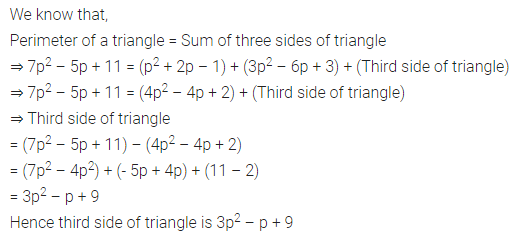# ML Aggarwal Class 8 Solutions for ICSE Maths Chapter 10 Algebraic Expressions and Identities Ex 10.1

## ML Aggarwal Class 8 Solutions for ICSE Maths Chapter 10 Algebraic Expressions and Identities Ex 10.1

Question 1.
Identify the terms, their numerical as well as literal coefficients in each of the following expressions:
(i) 12x2yz – 4xy2
(ii) 8 + mn + nl – lm
Solution:Question 2.
Identify monomials, binomials, and trinomials from the following algebraic expressions :
(i) 5p × q × r2
(ii) 3x2 + y ÷ 2z
(iii) -3 + 7x2
(iv) $$\frac{5 a^{2}-3 b^{2}+c}{2}$$
(v) 7x5 – $$\frac{3 x}{y}$$
(vi) 5p ÷ 3q – 3p2 × q2
Solution: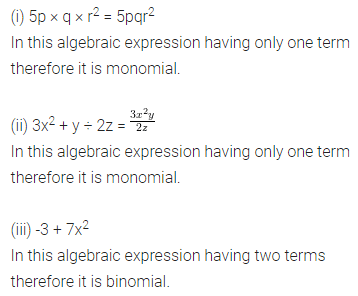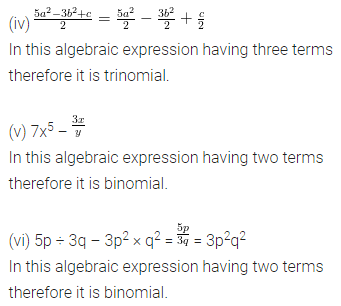Question 3.
Identify which of the following expressions are polynomials. If so, write their degrees.
(i) $$\frac{2}{5}$$x4 – $$\sqrt{3}$$x2 + 5x – 1
(ii) 7x3 – $$\frac{3}{x^{2}}$$ + $$\sqrt{5}$$
(iii) 4a3b2 – 3ab4 + 5ab + $$\frac{2}{3}$$
(iv) 2x2y – $$\frac{3}{x y}$$ + 5y3 + $$\sqrt{3}$$
Solution: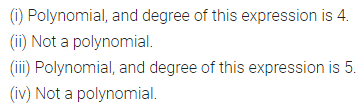Question 4.
(i) ab – bv, bv – ca, ca – ab
(ii) 5p2q2 + 4pq + 7,3 + 9pq – 2p2q
(iii) l2 + m2 + n2, lm + mn, mn + nl, nl + lm
(iv) 4x3 – 7x2 + 9, 3x2 – 5x + 4, 7x3 – 11x + 1, 6x2 – 13x
Solution:Question 5.
Subtract:
(i) 8a + 3ab – 2b + 7 from 14a – 5ab + 7b – 5
(ii) 8xy + 4yz + 5zx from 12xy – 3yz – 4zx + 5xyz
(iii) 4p2q – 3pq + 5pq2 – 8p + 7q -10 from 18 – 3p – 11q + 5pq – 2pq2 + 5p2q
Solution: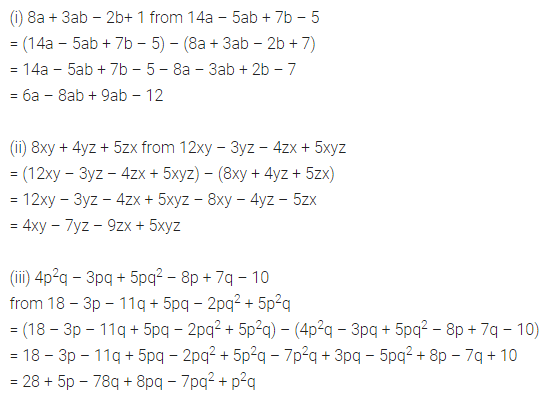Question 6.
Subtract the sum of 3x2 + 5xy + 7y2 + 3 and 2x2 – 4xy – 3y2 + 7 from 9x2 – 8xy + 11y2
Solution:Question 7.
What must be subtracted from 3a2 – 5ab – 2b2 – 3 to get 5a2 – 7ab – 3b2 + 3a ?
Solution:Question 8.
The perimeter of a triangle is 7p2 – 5p + 11 and two of its sides are p2 + 2p – 1 and 3p2 – 6p + 3. Find the third side of the triangle.
Solution: